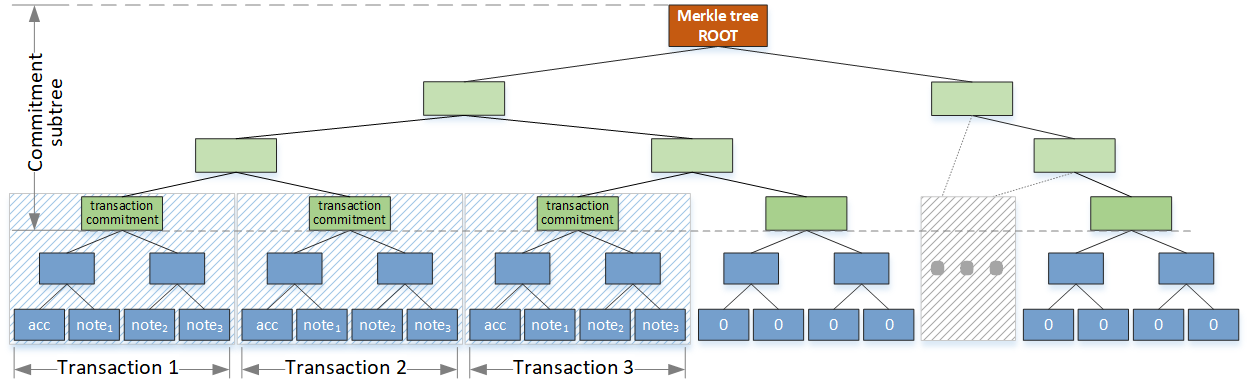# ZeroPool Merkle TreeThe merkle tree in the ZeroPool solution is used to link and store encrypted transaction data (accounts and notes) within a strict sequence. The accounts and note hashes are placed in the tree leaves.

The Merkle tree leaves and nodes contain hashes. Each node is calculated as a hash of two child nodes. Each leaf depends on its type (account and note leaves). The Poseidon function is used to calculate hashes with the appropriate parameters.

The full Merkle tree can be divided into a transaction subtree and a commitment subtree (with transaction commitments as leaves).

The commitment subtree ensures the correct transaction sequence without transaction data processing.

The transaction subtree links underlying data (accounts and notes) within a single operation. The transaction subtree's root is called the "transaction commitment." The commitments are using as commitment subtree leaves. Each transaction subtree is built using the corresponding memo block.

caution

#### Merkle tree height​

Note the tree in the picture above is not on its actual height. It's just a simple representation.

The ZeroPool solution uses a Merkle tree with a total height of 48 (transaction subtree with a height of 7 and commitment subtree with a height of 41).

Due to this the height transaction supports up to 128 leaves (a single account plus 127 notes) and the tree supports up to $2^{41} \approx 2.2 * 10^{12}$ transactions

According to the scheme above, a new transaction determines a new transaction subtree which will be added to the full Merkle tree. Adding a new transaction affects the Merkle tree root. So the Merkle tree root determines a current tree state.

The pool contract holds the current leaves count (the number of transactions multiplied by 128) and the Merkle tree roots for all transactions.